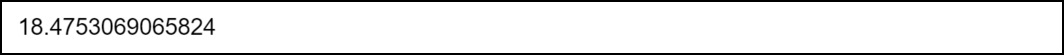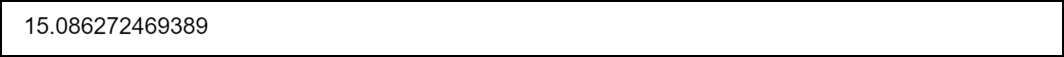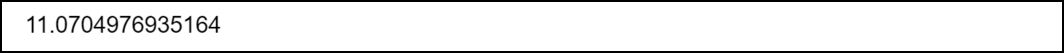# How to Find the Chi-Square Critical Value in R

• Last Updated : 28 Mar, 2022

In this article, we are going to see how to find the Chi-Square Critical Value in R programming language.

When the Chi-Square test is conducted, we get test statistics as an outcome. In order to find out whether the results of the Chi-Square are statistically significant, the test statistic is compared with the Chi-Square critical value. If the outcome of the test-statistic comes out to be greater than the Chi-Square statistic, the results of the test are considered statistically significant.

In order to Chi-Square critical value, we need the following data beforehand:

• A significance level
• Degrees of freedom

## Determining Chi-Square critical value in R

In order to determine Chi-Square critical value, R provides us qchisq() function that has the following syntax:

Syntax: qchisq(p, df, lower.tail=TRUE)

Parameters:

•  p: The significance level to use
• df: The degrees of freedom
• lower.tail = TRUE: Then the probability to the left of p in the F distribution is returned
• lower.tail = FALSE: Then the probability to the right is returned.
• Note that by default is TRUE.

Return Type: Returns the critical-value from the Critical-Square distribution

Let us consider an example in which we need to determine the Chi-Square critical value for the following data:

• df = 7
• significance level = 0.01

## R

 `# Determine the Chi-Square critical value ` `qchisq``(p = .01, df = 7, lower.tail = ``FALSE``)`

Output:Hence, the Chi-Square critical value for a significance level of 0.01 and degrees of freedom = 7 comes out to be equal to 18.475. Hence, if the Chi-Square test statistic comes out to be greater than 18.475 then the results of the test would be considered statistically significant.

## Relation of alpha and chi-square statistic

Alpha and chi-square critical values are inversely proportional to each other. In other words, larger critical values lead to smaller alpha values. Let the significance level be 0.01 and the degree of freedom be 5. Now, let’s compute the Chi-Square critical value:

Example 1:

## R

 `# Determine Chi-Square critical value ` `qchisq``(p = .01, df = 5, lower.tail = ``FALSE``)`

Output:Now let the significance level be having the same degree of freedom as taken in the previous example (that is 5) but the significance level taken is 0.05:

Example 2:

## R

 `# Determine Chi-Square critical value ` `qchisq``(p = .05, df = 5, lower.tail = ``FALSE``)`

Output:As you can see in the output, by increasing the significance level from 0.01 to 0.05 the Chi-Square critical value decreased from 15.086 to 11.070.

My Personal Notes arrow_drop_up
Recommended Articles
Page :